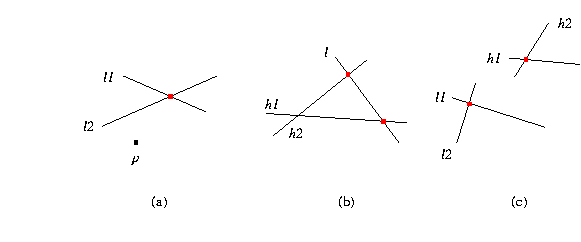## Kernel::CompareY_2

A model for this must provide:

 Comparison_result fo.operator() ( Kernel::Point_2 p, Kernel::Point_2 q) Compares the Cartesian y-coordinates of points p and qComparison_result fo.operator() ( Kernel::Point_2 p, Kernel::Line_2 l1, Kernel::Line_2 l2) compares the y-coordinates of p and the intersection of lines l1 and l2, see (a) in the figure above. Comparison_result fo.operator() ( Kernel::Line_2 l, Kernel::Line_2 h1, Kernel::Line_2 h2) compares the y-coordinates of the intersection of line l with line h1 and with line h2, see (b) in the figure above. Comparison_result fo.operator() ( Kernel::Line_2 l1, Kernel::Line_2 l2, Kernel::Line_2 h1, Kernel::Line_2 h2) compares the y-coordinates of the intersection of lines l1 and l2 and the intersection of lines h1 and h2 , see (c) in the figure above.